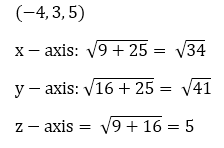×#### Thank you for registering.

One of our academic counsellors will contact you within 1 working day.

Click to Chat

1800-1023-196

+91-120-4616500

CART 0

• 0

MY CART (5)

Use Coupon: CART20 and get 20% off on all online Study Material

ITEM
DETAILS
MRP
DISCOUNT
FINAL PRICE
Total Price: Rs.

There are no items in this cart.
Continue Shopping• Complete JEE Main/Advanced Course and Test Series
• OFFERED PRICE: Rs. 15,900
• View Details

```Chapter 28: Introduction to 3D coordinate geometry – Exercise 28.1

Introduction to 3D coordinate geometry – Exercise – 28.1 – Q.1(i)

Ans.

All are positive. So octant is XOYZ

Introduction to 3D coordinate geometry – Exercise – 28.1 – Q.1(ii)

Ans.

X is negative and rest are positive, so octant is X’OYZ

Introduction to 3D coordinate geometry – Exercise – 28.1 – Q.1(iii)

Ans.

Y is negative and rest are positive, so octant is XOY’Z

Introduction to 3D coordinate geometry – Exercise – 28.1 – Q.1(iv)

Ans.

Z is negative and rest are positive, so octant is XOYZ’

Introduction to 3D coordinate geometry – Exercise – 28.1 – Q.1(v)

Ans.

X and Y are negative and Z is positive, so octant is X'OY'Z

Introduction to 3D coordinate geometry – Exercise – 28.1 – Q.1(vi)

Ans.

All are negative, so octant is X’OY’Z’

Introduction to 3D coordinate geometry – Exercise – 28.1 – Q.1(vii)

Ans.

Y and Z are negative, so octant is XOY’Z’

Introduction to 3D coordinate geometry – Exercise – 28.1 – Q.1(viii)

Ans.

X and Z are negative, so octant is X’OYZ’

Introduction to 3D coordinate geometry – Exercise – 28.1 – Q.2(i)

Ans.

YZ plane is x-axis, so sign of x will be changed. So answer is (2, 3, 4)

Introduction to 3D coordinate geometry – Exercise – 28.1 – Q.2(ii)

Ans.

XZ plane is y-axis, so sign of y will be changed. So answer is (-5, -4, -3)

Introduction to 3D coordinate geometry – Exercise – 28.1 – Q.2(iii)

Ans.

XY-plane is z-axis, so sign of Z will change. SO answer is (5, 2, 7)

Introduction to 3D coordinate geometry – Exercise – 28.1 – Q.2(iv)

Ans.

XZ plane is y-axis, so sign of Y will change, So answer is (-5. 0. 3)

Introduction to 3D coordinate geometry – Exercise – 28.1 – Q.2(v)

Ans.

XY plane is Z-axis, so sign of Z will change So Answer is (-4, 0, 0)

Introduction to 3D coordinate geometry – Exercise – 28.1 – Q.3

Ans.

Vertices of cube are

(1, 0, -1) (1, 0, 4) (1, -5, -1)

(1, -5, 4)(-4, 0, -1)(-4, -5, -4)

(-4, -5, -1) (4, 0, 4)(1, 0, 4)

Introduction to 3D coordinate geometry – Exercise – 28.1 – Q.4

Ans.

3 – (-2) = 5, |0, -5| = =5,| – 1 – 4| = 5

5, 5, 5 are lengths of edges

Introduction to 3D coordinate geometry – Exercise – 28.1 – Q.5

Ans.

5 – 3 = 2, 0 – (- 2) = 2, 5 – 2 = 3

2, 2, 3 are lengths of edges.

Introduction to 3D coordinate geometry – Exercise – 28.1 – Q.6

Ans.Introduction to 3D coordinate geometry – Exercise – 28.1 – Q.7

Ans.

(-3, -2, -5) (-3, -2, 5) (3, -2. -5) (-3, 2, -5) (3, 2, 5) (3, 2, -5) (-3, 2, 5)

```### Course Features

• 728 Video Lectures
• Revision Notes
• Previous Year Papers
• Mind Map
• Study Planner
• NCERT Solutions
• Discussion Forum
• Test paper with Video Solution